# Solve multiplication equations formula Solve Multiplication Equations Solve

• Slides: 13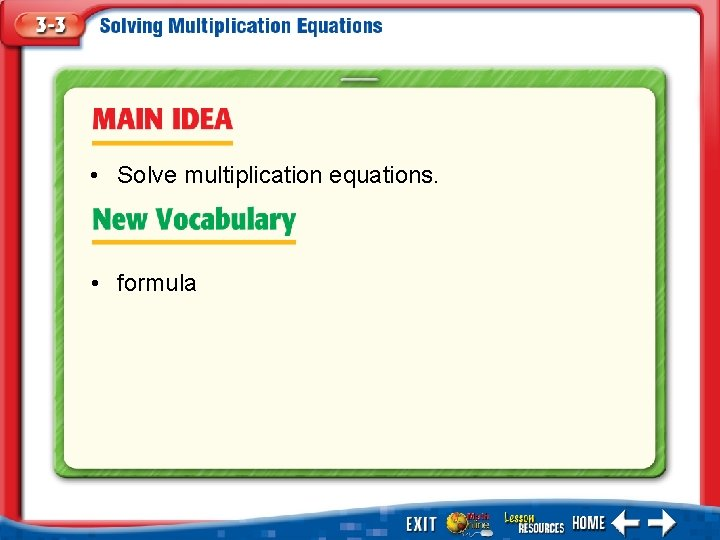• Solve multiplication equations. • formula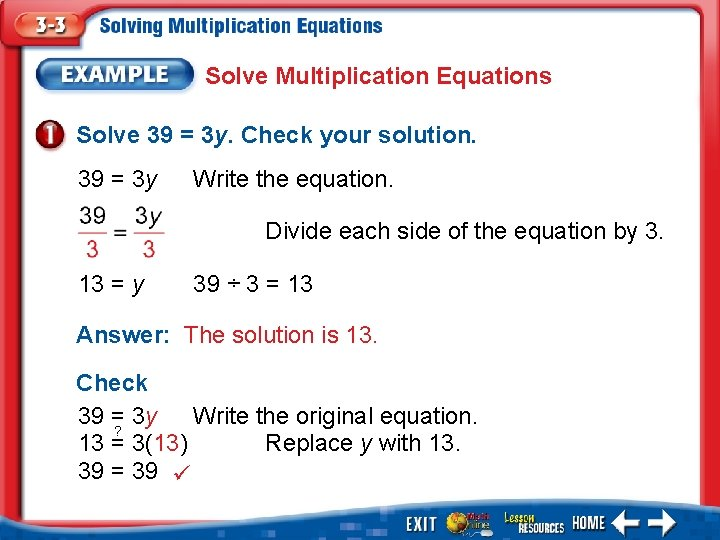Solve Multiplication Equations Solve 39 = 3 y. Check your solution. 39 = 3 y Write the equation. Divide each side of the equation by 3. 13 = y 39 ÷ 3 = 13 Answer: The solution is 13. Check 39 =? 3 y Write the original equation. 13 = 3(13) Replace y with 13. 39 = 39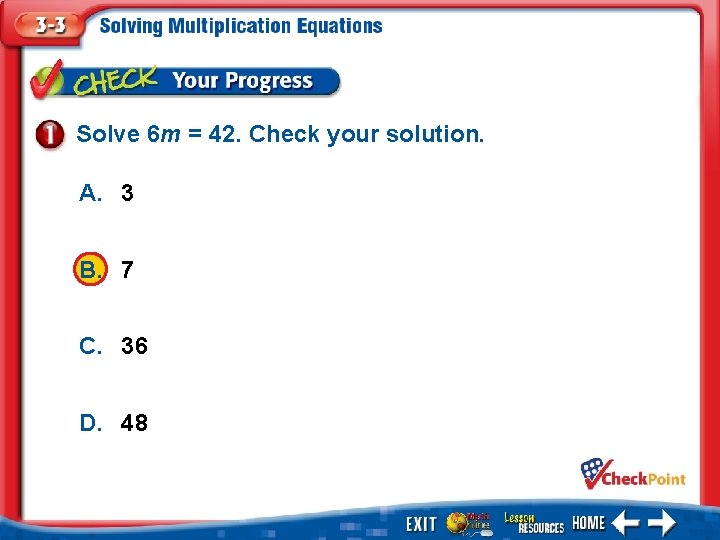Solve 6 m = 42. Check your solution. A. 3 B. 7 C. 36 D. 48 1. 2. 3. 4. A B C DSolve Multiplication Equations Solve – 4 z = 60. Check your solution. – 4 z = 60 Write the equation. Divide each side of the equation by – 4. z = 15 60 ÷ (– 4) = – 15 Answer: The solution is – 15. Check – 4 z = 60 ? – 4(– 15) = 60 60 = 60 Write the original equation. Replace z with – 15.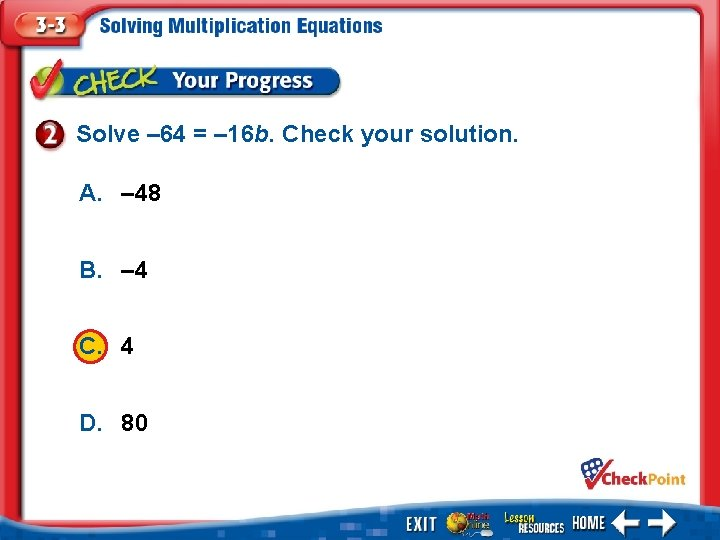Solve – 64 = – 16 b. Check your solution. A. – 48 B. – 4 C. 4 D. 80 1. 2. 3. 4. A B C DMAIL Serena went to the post office to mail some party invitations. She had \$6. 15. If each invitation needed a \$0. 41 stamp, how many invitations could she mail? Words Total is equal to cost of each stamp times number of invitations mailed. Variable Let n represent the number of invitations mailed. Equation 6. 15 = 0. 41 n6. 15 = equation. 0. 41 n Write the 15 = 6. 15 ÷ 0. 41 = 15 n Answer: Serena could mail 15 invitations.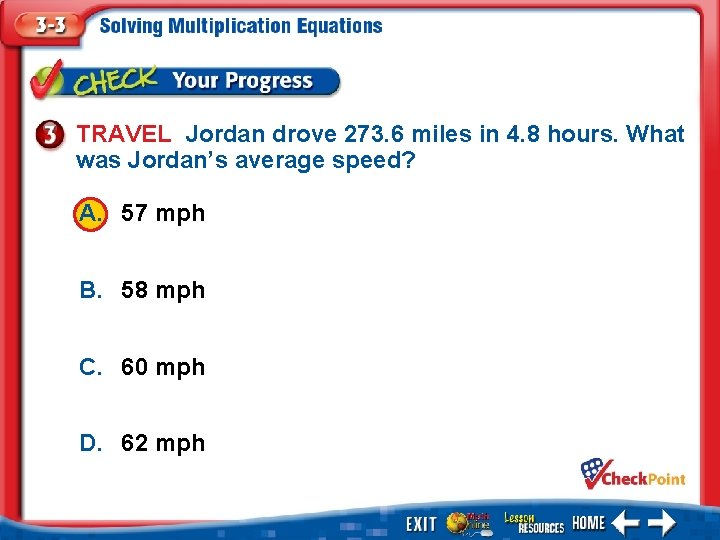TRAVEL Jordan drove 273. 6 miles in 4. 8 hours. What was Jordan’s average speed? A. 57 mph B. 58 mph C. 60 mph D. 62 mph 1. 2. 3. 4. A B C DSWIMMING Ms. Wang swims at a speed of 0. 6 mph. At this rate, how long will it take her to swim 3 miles? You are asked to find the time t it will take to travel a distance d of 3 miles at a rate r of 0. 6 mph. d = rt 3 = 0. 6 t Answer: It would take Ms. Wang 5 hours to swim 3 miles.COOKIES Debbie spends \$6. 85 on cookies at the bakery. The cookies are priced at \$2. 74 per pound. How many pounds of cookies did Debbie buy? A. 1. 75 pounds B. 2. 5 pounds C. 2. 75 pounds D. 4. 11 pounds 1. 2. 3. 4. A B C D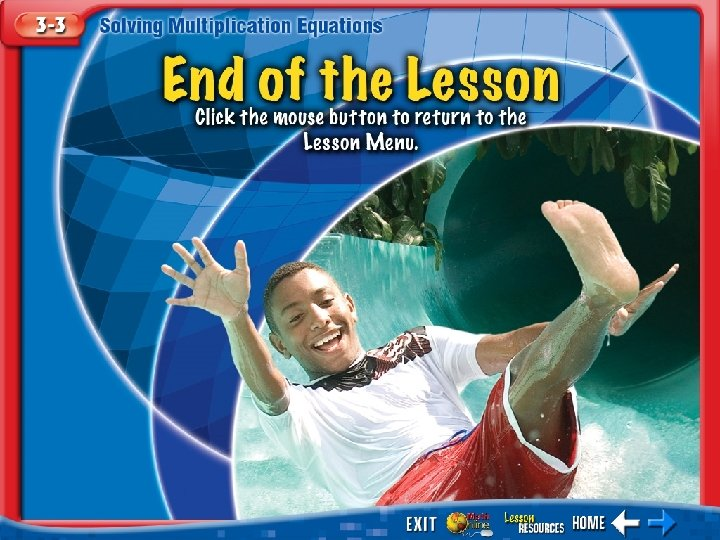End of the Lesson